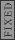GR 8677927796770177 | # Login | Register

GR9277 #84
Problem
 GREPhysics.NET Official Solution Alternate Solutions
\prob{84}
An electron oscillates back and forth along the + and -x axes, consequently emitting electromagnetic radiation. Which of the following statements concerning the radiation is NOT true?

1. The total rate of radiation of energy into all directions is proportional to the square of the electron's acceleration.
2. The total rate of radiation of energy into all directions is proportional to the square of the electron's charge.
3. Far from the electron, the rate at which radiated energy crosses a perpendicular unit area decreases as the inverse square of the distance from the electron
4. Far from the electron, the rate at which radiated energy crosses a perpendicular unit area is a maximum when the unit area is located on the + or - x-axes
5. Far from the electron, the radiated energy is carried equally by the transverse electric and the transverse magnetic fields.

Electromagnetism}Accelerating Charges

Elimination time:

(A) By the Larmor formula, one has , where q is the charge and a is the acceleration. Since the charge is constant, this choice is true.

(B) This is also true by Larmor's formula.

(C) True. The energy radiated through a perpendicular unit area is given by the Poynting vector. and far away. Also, less rigorously, one can arrive at the same result from recalling the surface area of a sphere, , and thus any term in proportional to will yield a finite, thus acceptable answer. (Thanks to the user astro_allison for this pointer. See p460ff of Griffiths, Introduction to Electrodynamics, 3rd Edition for more details.)

(D) False. It's a minimum in the plane.

(E) True, since far from the electron the field behaves as plane waves, with and both 0.Alternate Solutions
 Herminso2009-09-22 14:16:29 Remember the Larmor Formula , thus (A) and (B) are right. Now, for a electric dipole we have: and You see then that far from the electron, the radiated energy is carried equally by the transverse electric and the transverse magnetic fields.Then (E) is true. To see if the rate at which radiated energy crosses a perpendicular unit area decreases as the inverse square of the distance from the electron, look for the Pointing vector: , thus (C) is also true. But (D) is false if from the expresion above for the electric field you can see that it ahve a minimum at , just the x-axes. Reply to this commenttn
2018-01-23 02:39:21
If, and , then wouldn\'t ? I think the answer to this problem is contingent upon being able to model the oscillating electron as a Hertzian dipole, which generates a field . Then we can conclude .
 tn2018-01-23 02:43:54 TYPO! comment above should read:\r\n\r\nIf, and , then wouldn\'t ? I think the answer to this problem is contingent upon being able to model the oscillating electron as a Hertzian dipole, which generates a field . Then we can conclude .
 tn2018-01-23 02:46:39 TYPO! comment above should read:\r\n\r\nIf, and , then wouldn\'t ? I think the answer to this problem is contingent upon being able to model the oscillating electron as a Hertzian dipole, which generates a field . Then we can conclude .Herminso
2009-09-22 14:16:29
Remember the Larmor Formula , thus (A) and (B) are right.

Now, for a electric dipole we have:

and

You see then that far from the electron, the radiated energy is carried equally by the transverse electric and the transverse magnetic fields.Then (E) is true.

To see if the rate at which radiated energy crosses a perpendicular unit area decreases as the inverse square of the distance from the electron, look for the Pointing vector:

, thus (C) is also true.

But (D) is false if from the expresion above for the electric field you can see that it ahve a minimum at , just the x-axes.georgi
2007-08-27 00:10:23
i'm not sure i understand why d is false. What plane are we talking about? and why is that true?
 a19grey22008-11-02 14:33:05 Yosun is referring to the Y-Z plane in this problem. The maximum amount of energy radiated is along the x-axis (the axis of acceleration) and it is minimum perpendicular to the axis of acceleration).
 Crandor2010-04-08 19:13:17 Exactly backwards, a19grey2. Power radiated is minimum (0) along the axis of motion and maximum along the plane perpendicular to that axis.astro_allison
2005-11-26 04:52:46
(c) is true because energy (radiated energy here) is proportional to the inverse square of the distance. I know this is more or less exactly what the problem states; just remember that for and electron, , where r is the distance.
 Andresito2006-03-29 15:09:21 @ astro_allison, thank you for the reference. @ Yosun, correct page is "460"astro_allison
2005-11-26 04:15:07
 astro_allison2005-11-26 04:15:37 (from griffiths em)
 yosun2005-11-26 18:14:17 astro_allison: thanks for the typo-alert regarding Larmor's formula, as well as the pointer to check Griffiths.LaTeX syntax supported through dollar sign wrappers $, ex.,$\alpha^2_0$produces . type this... to get...$\int_0^\infty\partial\Rightarrow\ddot{x},\dot{x}\sqrt{z}\langle my \rangle\left( abacadabra \right)_{me}\vec{E}\frac{a}{b}\$